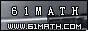Today is . WELCOME: huohai3983/44663  Home | Reg Login | All user | setHomePage | BookmarkHome | Math Test Online | Addition | Subtraction | Multiplication | Division | Time | Worksheet(JPG/PDF) | CAI | Flash Games |Recommendation articleArticle searchHome--maths--Second Grade--Adding Two Digit Numbers Without Renaming

Adding Two Digit Numbers Without Renaming

How to add two two-digit numbers (for example 45 + 53).

• Place one number above the other number so that the tens' place digits and ones' place digits are lined up. Draw a line under the bottom number.
```45
53
```
• Add the two ones' place digits (5 + 3 = 8).
```45
53
8
```
• Add the numbers in the tens' place column (4 + 5 = 9) and place the answer below the line and to the left of the ones' place sum.
``` 45
53
98```

#### Find the Sum of the two Numbers

 +     =

2006-10-02 20:08:56
Viewed 4113 times CloseGreatmathsites

### Translate

 Thank you to online math channels' Mathematics Department for hosting these lessons. Copyright © 2006 - 2008 GZtrain Inc. All Rights Reserved